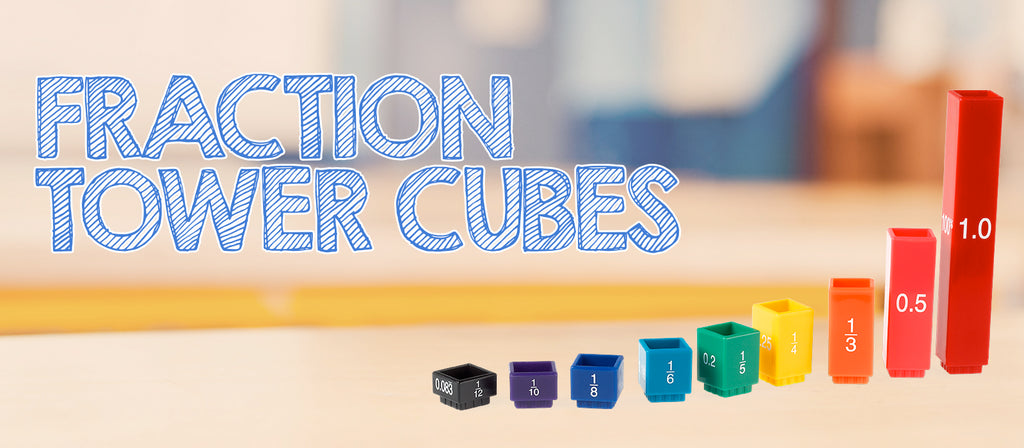# Learning with Fraction Tower CubesFraction Tower Cubes represent different fractions for instructive comparison. You can expand the possibilities of teaching fractions and equivalency with these proportionally sized cubes. Teach students how to compare and construct fractions, work with mixed numbers, and add/subtract fractions with like and unlike denominators.

Students should be given the opportunity to become comfortable and familiar with Fraction Tower Cubes by creatively manipulating them. Encourage students to use the cubes to create patterns and designs. Challenge students to talk about the Fraction Tower Cubes and how they relate to one another.

Rainbow Fraction® Tower Cubes represent different fractions for instructive comparison. Rainbow Fraction Tower Cubes represent a whole, halves, thirds, fourths, fifths, sixths, eighths, tenths, and twelfths. Each tile is broken into equal fractional parts and uses the same-sized whole.

Shop Fraction Tower Cubes Now!

Skills

• - Add and Subtract Fractions
• - Equivalences
• - Mixed Numbers
• - Comparing Fractions
• - Common Denominators
• - Dividing Fractions
• - Ratios

Shop Fraction Tower Cubes Now!

• Raana Smith